2019-02-03 14:54:15 qq_42505705 阅读数 1379
• ###### webgl基础篇视频教程-坚如磐石

26464 人正在学习 去看看 张立铜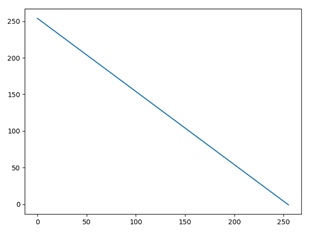import cv2
import numpy as np

reverse_img = 255 - img

cv2.imshow('srcimg',img)
cv2.imshow('reverse_img',reverse_img)
cv2.waitKey(0)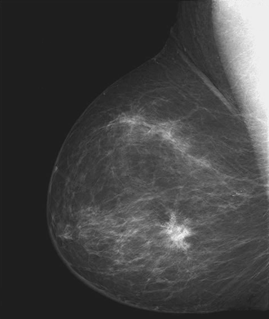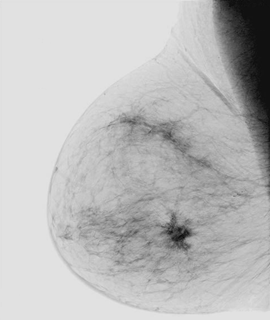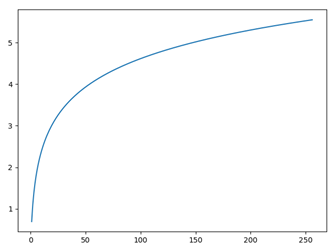import cv2
import math
import numpy as np

def logTransform(c,img):

#3通道RGB
'''h,w,d = img.shape,img.shape,img.shape
new_img = np.zeros((h,w,d))
for i in range(h):
for j in range(w):
for k in range(d):
new_img[i,j,k] = c*(math.log(1.0+img[i,j,k]))'''

#灰度图专属
h,w = img.shape, img.shape
new_img = np.zeros((h, w))
for i in range(h):
for j in range(w):
new_img[i, j] = c * (math.log(1.0 + img[i, j]))

new_img = cv2.normalize(new_img,new_img,0,255,cv2.NORM_MINMAX)

return new_img

#替换为你的图片路径

log_img = logTransform(1.0,img)
cv2.imshow('log_img',log_img)
cv2.imwrite(r'C:\Users\xxx\Desktop\Fourier spectrum2.jpg',log_img)
cv2.waitKey(0)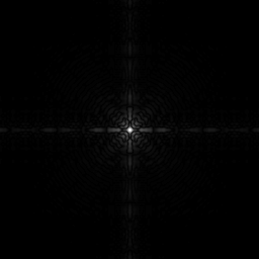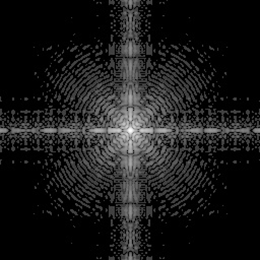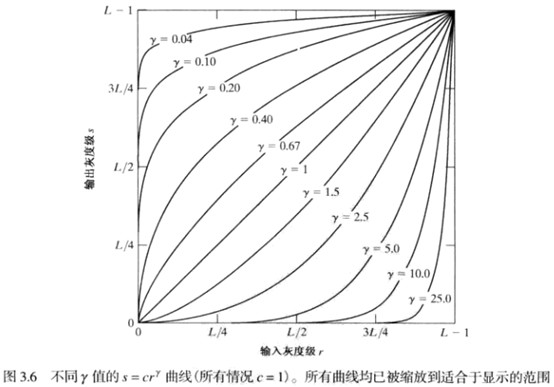import math
import numpy as np
import cv2

def gammaTranform(c,gamma,image):
h,w,d = image.shape,image.shape,image.shape
new_img = np.zeros((h,w,d),dtype=np.float32)
for i in range(h):
for j in range(w):
new_img[i,j,0] = c*math.pow(image[i, j, 0], gamma)
new_img[i,j,1] = c*math.pow(image[i, j, 1], gamma)
new_img[i,j,2] = c*math.pow(image[i, j, 2], gamma)
cv2.normalize(new_img,new_img,0,255,cv2.NORM_MINMAX)
new_img = cv2.convertScaleAbs(new_img)

return new_img

new_img = gammaTranform(1,2.5,img)

cv2.imshow('x',new_img)
cv2.imwrite(r'C:\Users\xxx\Desktop\gray_2.5.jpg',new_img)
cv2.waitKey(0)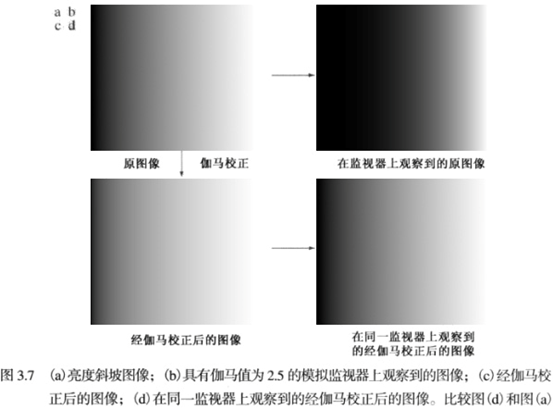γ<1 增强亮度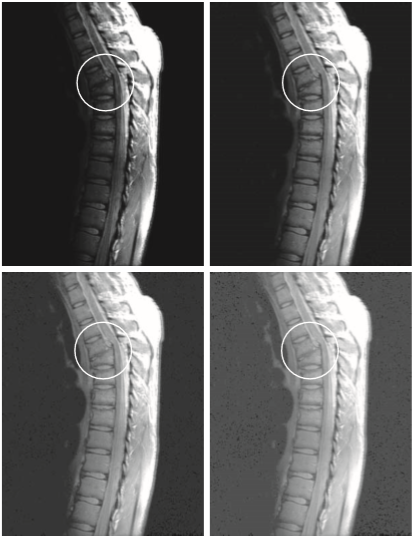γ>1 增强暗度2010-05-23 14:49:00 yangfenghero 阅读数 3459
• ###### webgl基础篇视频教程-坚如磐石

26464 人正在学习 去看看 张立铜

幂次变换的基本表达式为：y=cxr+b

其中cr均为正数。与对数变换相同，幂次变换将部分灰度区域映射到更宽的区域中。当r=1时，幂次变换转变为线性变换。

(1)  当r<0时，变换函数曲线在正比函数上方。此时扩展低灰度级，压缩高灰度级，使图像变亮。这一点与对数变换十分相似。

(2)  当r>0时，变换函数曲线在正比函数下方。此时扩展高灰度级，压缩低灰度级，使图像变暗。

代码如下：

2019-11-14 20:11:58 qq_41398808 阅读数 124
• ###### webgl基础篇视频教程-坚如磐石

26464 人正在学习 去看看 张立铜

>1的值所生成的曲线和<1所生成的曲线的效果完全相反，当c==1时简化为了恒等变换。导入要使用的第三方库：

from PIL import Image
import numpy as np
import matplotlib.pyplot as plt

读取图片数据，并可视化：

img = Image.open('小亮点.jpg')
plt.axis('off')
plt.imshow(img)
plt.show()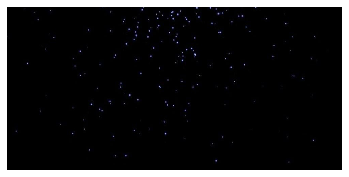图像数据转换为numpy数组：

img_data = np.array(img)

定义幂律变换函数：

def power_law_rollovers(img, func, c, b):
img_data = np.array(img)
a = np.shape(img_data)
new_img = []
for i in range(a):
new_row = []
for j in range(a):
data = list(img_data[i][j])
new_data = func(data, c, b)
new_row.append(np.array(new_data))
new_img.append(np.array(new_row))
return new_img
def power_law(data, c, b):
new_data = []
for k in data:
a = int(c*(k**b))
new_data.append(a)
return new_data

结果：

new_img1 = power_law_rollovers(img, power_law, 1, 0.8)
new_img2 = power_law_rollovers(img, power_law, 1, 0.6)
new_img3 = power_law_rollovers(img, power_law, 1, 0.4)
new_img4 = power_law_rollovers(img, power_law, 1, 0.3)

new_img1 = np.array(new_img1)
new_img2 = np.array(new_img2)
new_img3 = np.array(new_img3)
new_img4 = np.array(new_img4)

new_img1 = Image.fromarray(new_img1.astype('uint8')).convert('RGB')
new_img2 = Image.fromarray(new_img2.astype('uint8')).convert('RGB')
new_img3 = Image.fromarray(new_img3.astype('uint8')).convert('RGB')
new_img4 = Image.fromarray(new_img4.astype('uint8')).convert('RGB')

plt.figure(figsize=(25,60))
plt.subplot(221)
plt.axis('off')
gray1 = new_img1.convert('L')
plt.imshow(gray1, cmap='gray')
plt.subplot(222)
plt.axis('off')
gray2 = new_img2.convert('L')
plt.imshow(gray2, cmap='gray')
plt.subplot(223)
plt.axis('off')
gray3 = new_img3.convert('L')
plt.imshow(gray3, cmap='gray')
plt.subplot(224)
plt.axis('off')
gray4 = new_img4.convert('L')
plt.imshow(gray4, cmap='gray')
plt.show()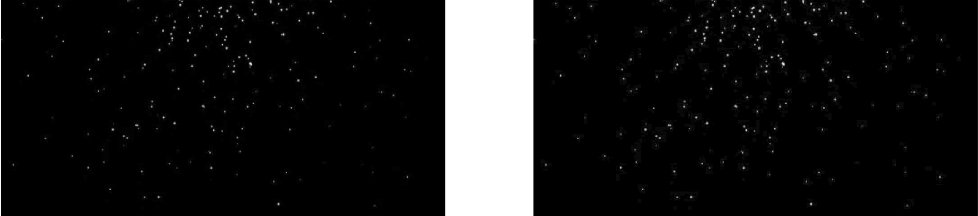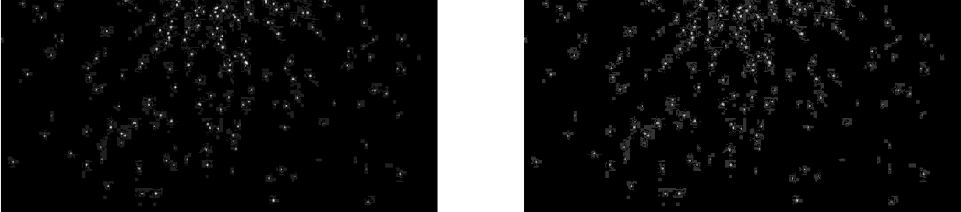2018-09-27 21:19:18 perry0528 阅读数 920
• ###### webgl基础篇视频教程-坚如磐石

26464 人正在学习 去看看 张立铜

### 对数变换：

CImg<int> SrcImg;
SrcImg.display();
cimg_forXY(SrcImg, x, y) {
SrcImg(x, y) = int(log(double(SrcImg(x, y)) + 1));
}
SrcImg.display();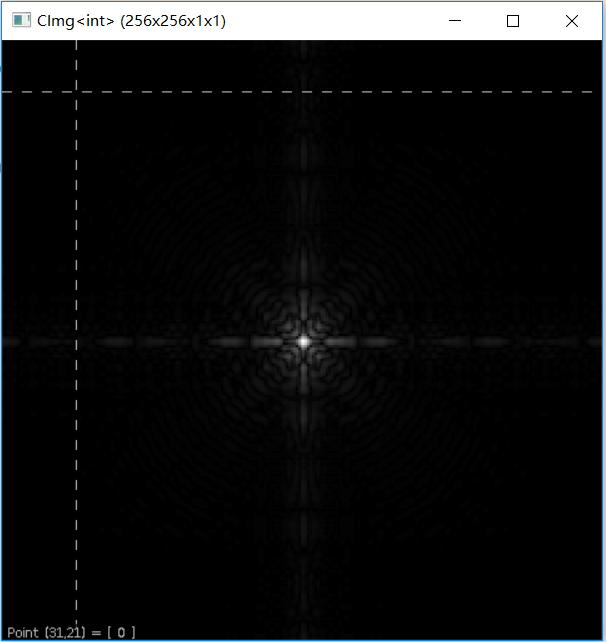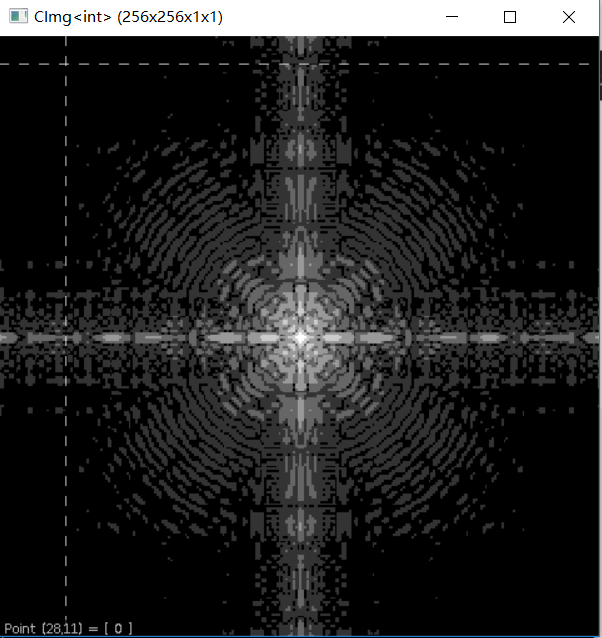### 幂律变换

CImg<int> SrcImg;
SrcImg.display();
double t = { 0.6,0.4,0.3 };
for (int i = 0; i < 3; i++) {
CImg<int> img = SrcImg;
cimg_forXY(img, x, y) {
img(x, y) = int(pow(double((img(x, y))), t[i]));
}
img.display();
}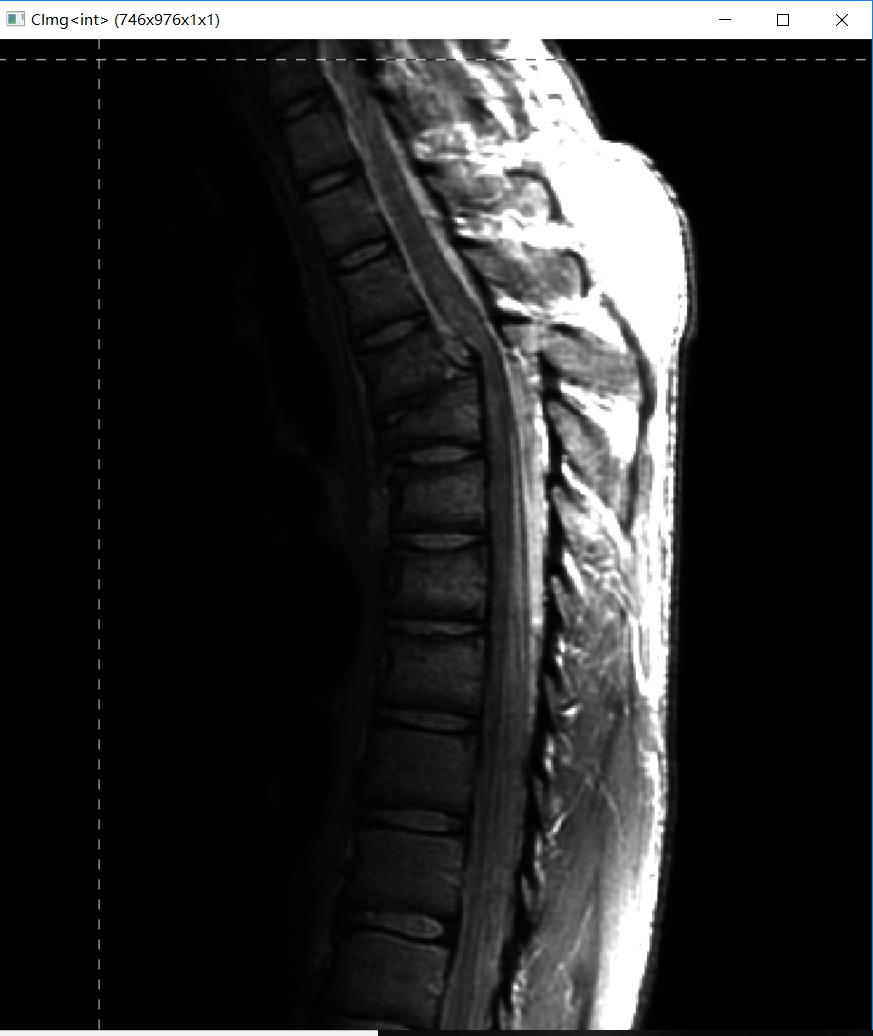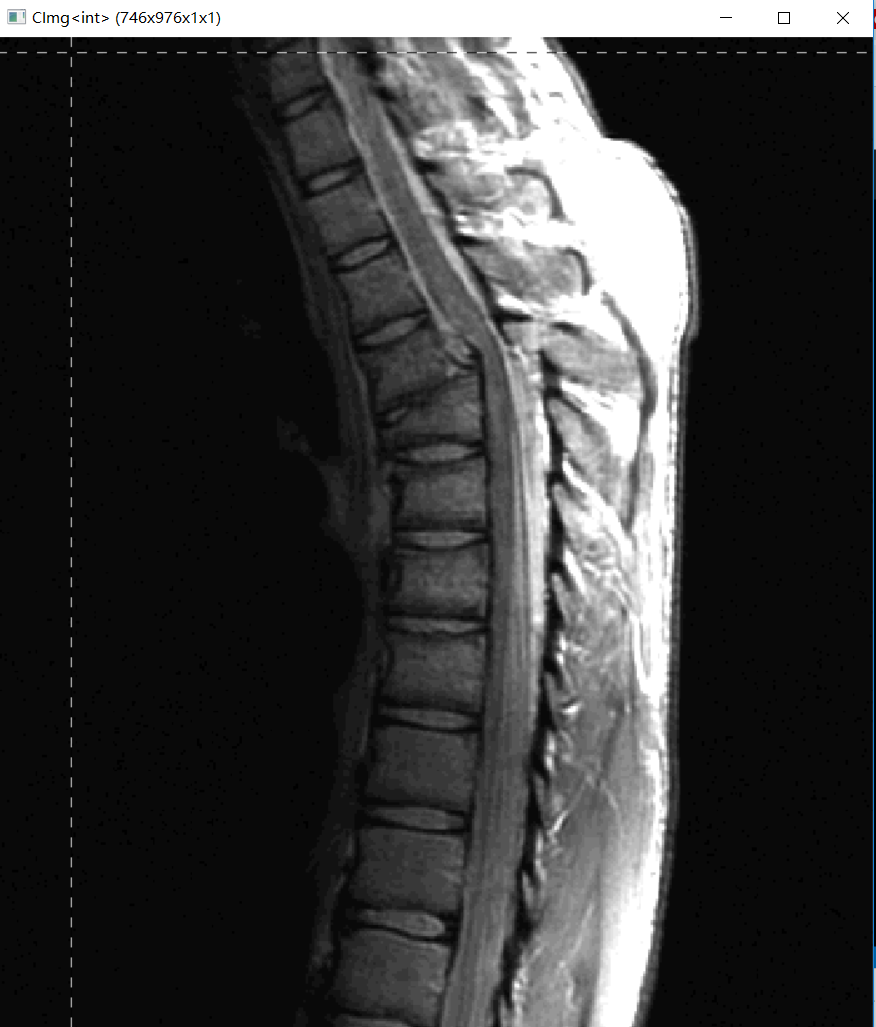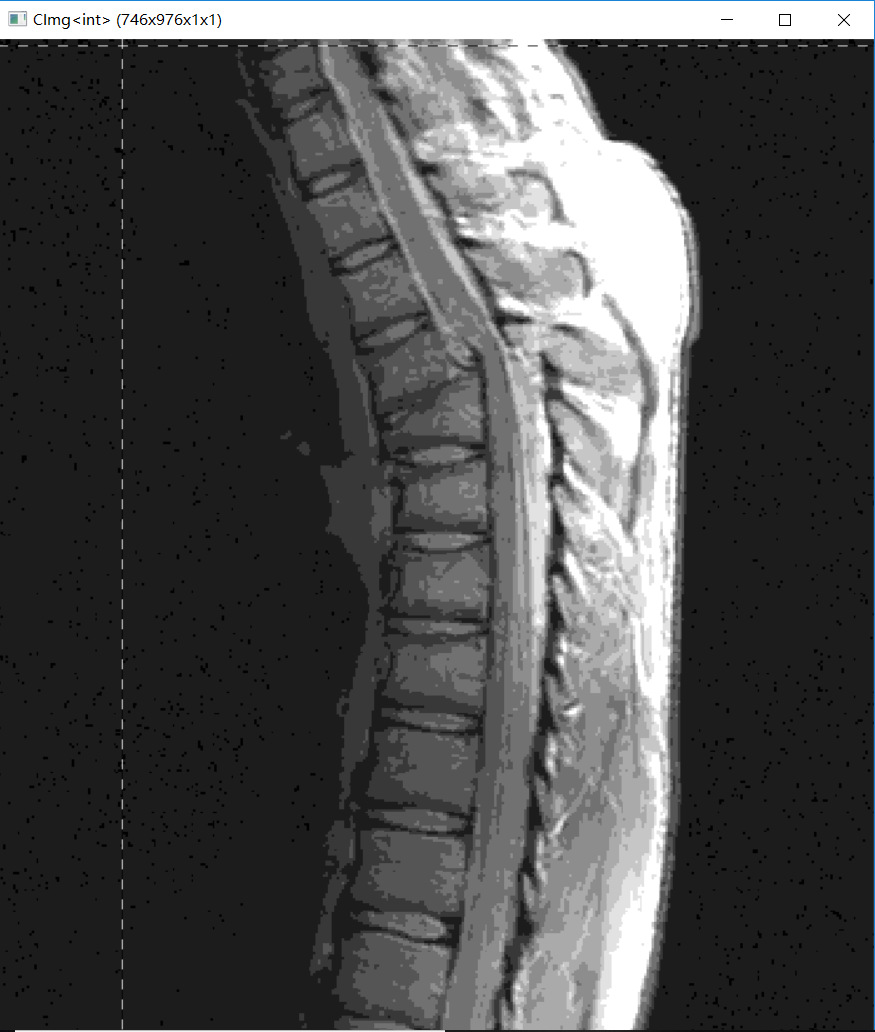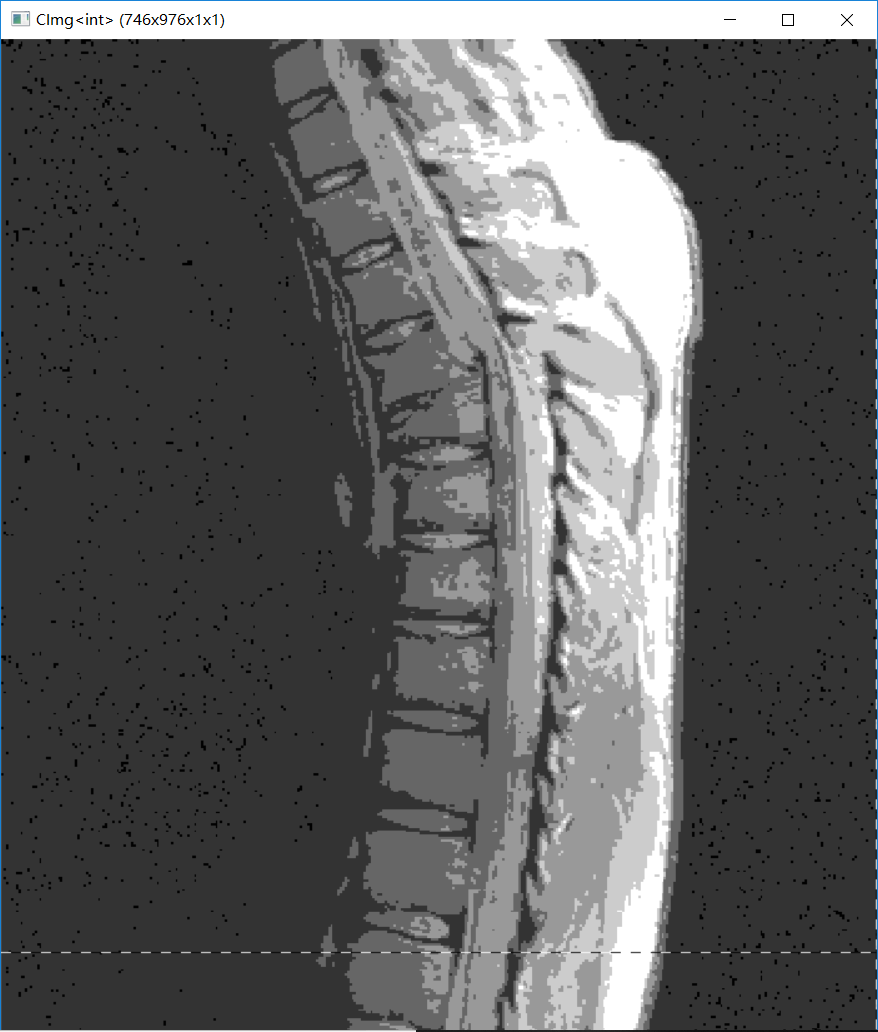### 比特面分层

CImg<int> img;
img.display("原图");
int w = img.height();
int h = img.width();
CImg<int> p1(h,w,1,1), p2(h, w, 1, 1), p3(h, w, 1, 1), p4(h, w, 1, 1), p5(h, w, 1, 1), p6(h, w, 1, 1), p7(h, w, 1, 1), p8(h, w, 1, 1);
cimg_forXY(img, x, y) {
int num = img(x, y);
int *bit = binary(num);
p1(x, y) = fx(bit); p2(x, y) = fx(bit); p3(x, y) = fx(bit); p4(x, y) = fx(bit);
p5(x, y) = fx(bit); p6(x, y) = fx(bit); p7(x, y) = fx(bit); p8(x, y) = fx(bit);
}
p8.display("第八层比特图");
p7.display("第七层比特图");
p6.display("第六层比特图");
p5.display("第五层比特图");
p4.display("第四层比特图");
p3.display("第三层比特图");
p2.display("第二层比特图");
p1.display("第一层比特图");
}
//用于对图片进行黑白的赋值
int fx(int n) {
if (n == 1)
return 255;
else
return 0;
}
//用于进行二进制转化的函数
int* binary(int num) {
int *bit;
bit = new int;
for (int i = 0; i < 8; i++) {
bit[i] = 0;
}
int i = 0;
while (num) {
bit[i] = num % 2;
num /= 2;
i++;
}
return bit;
}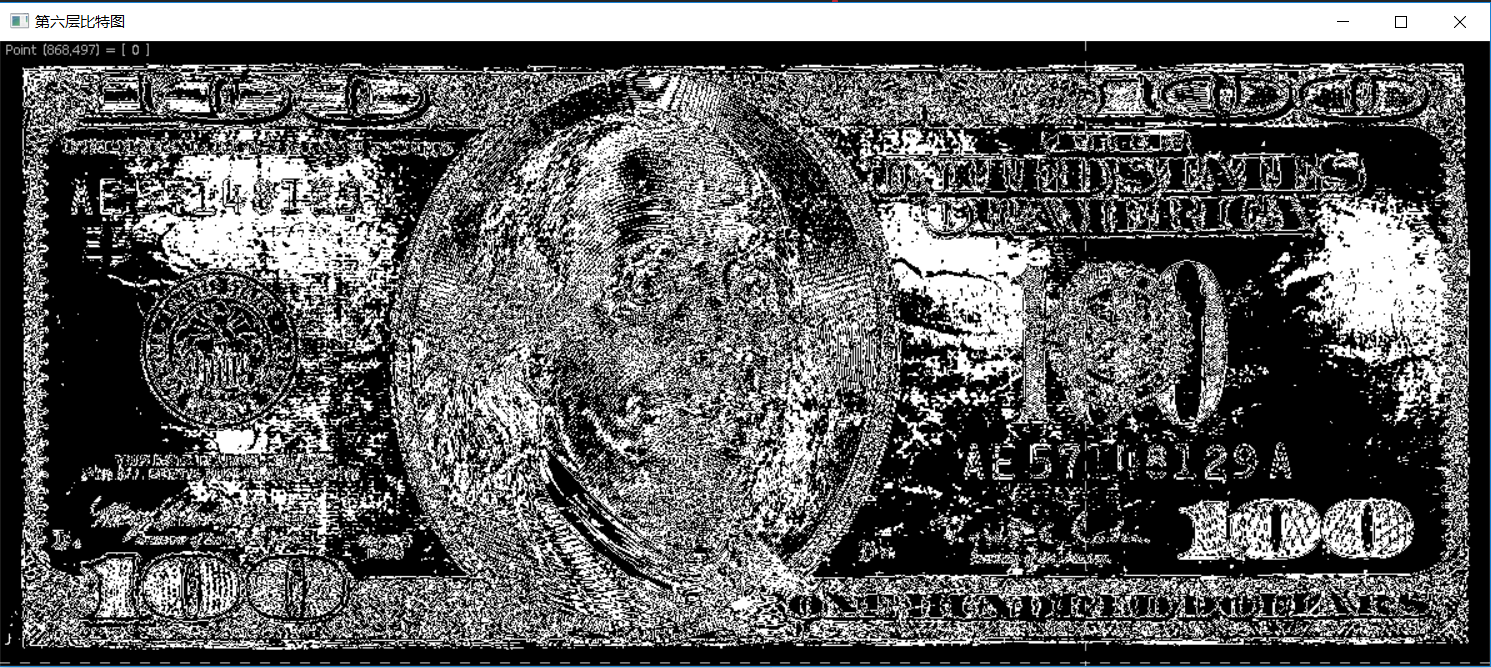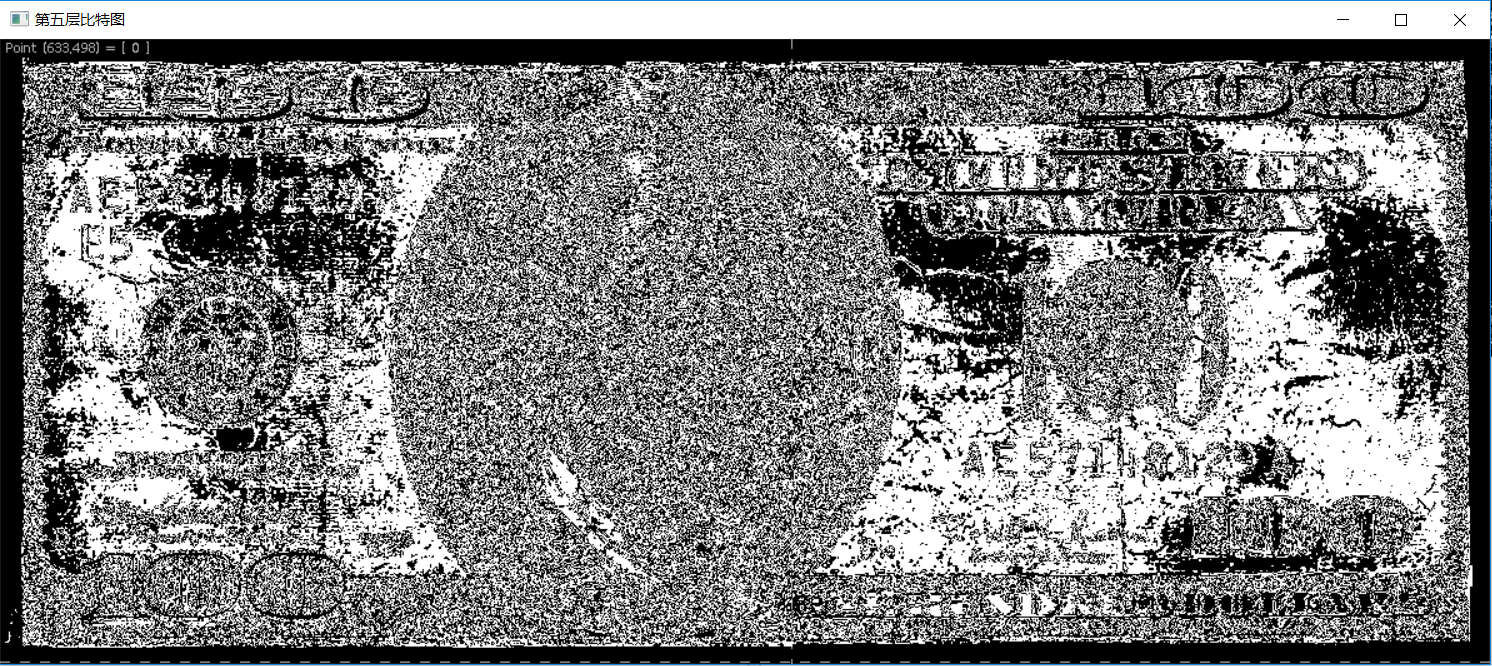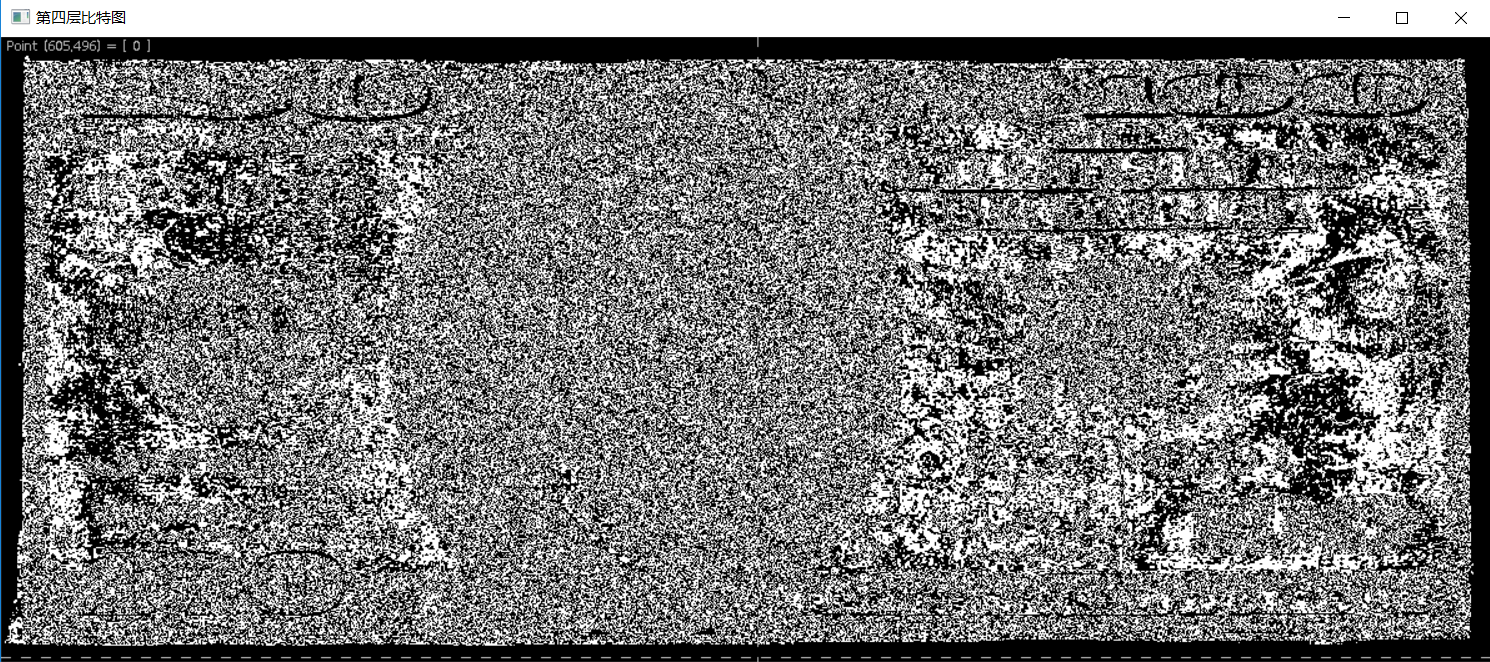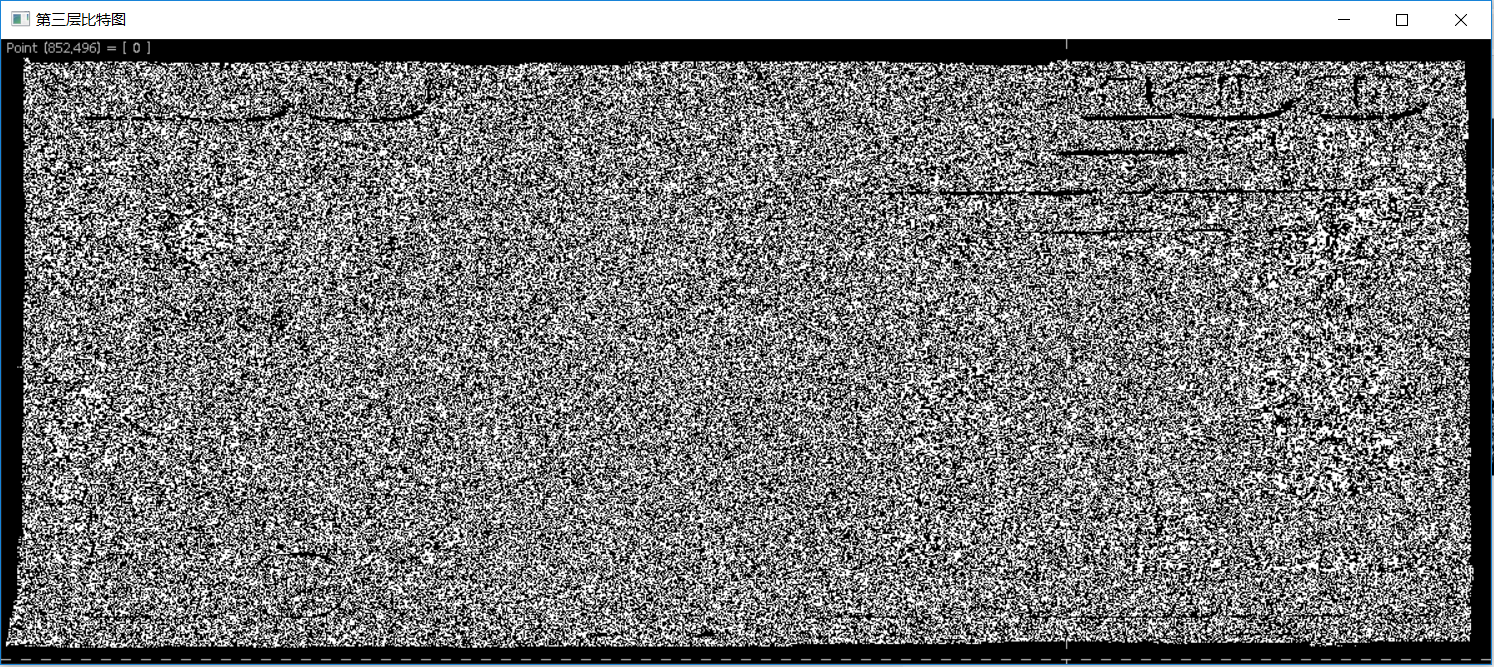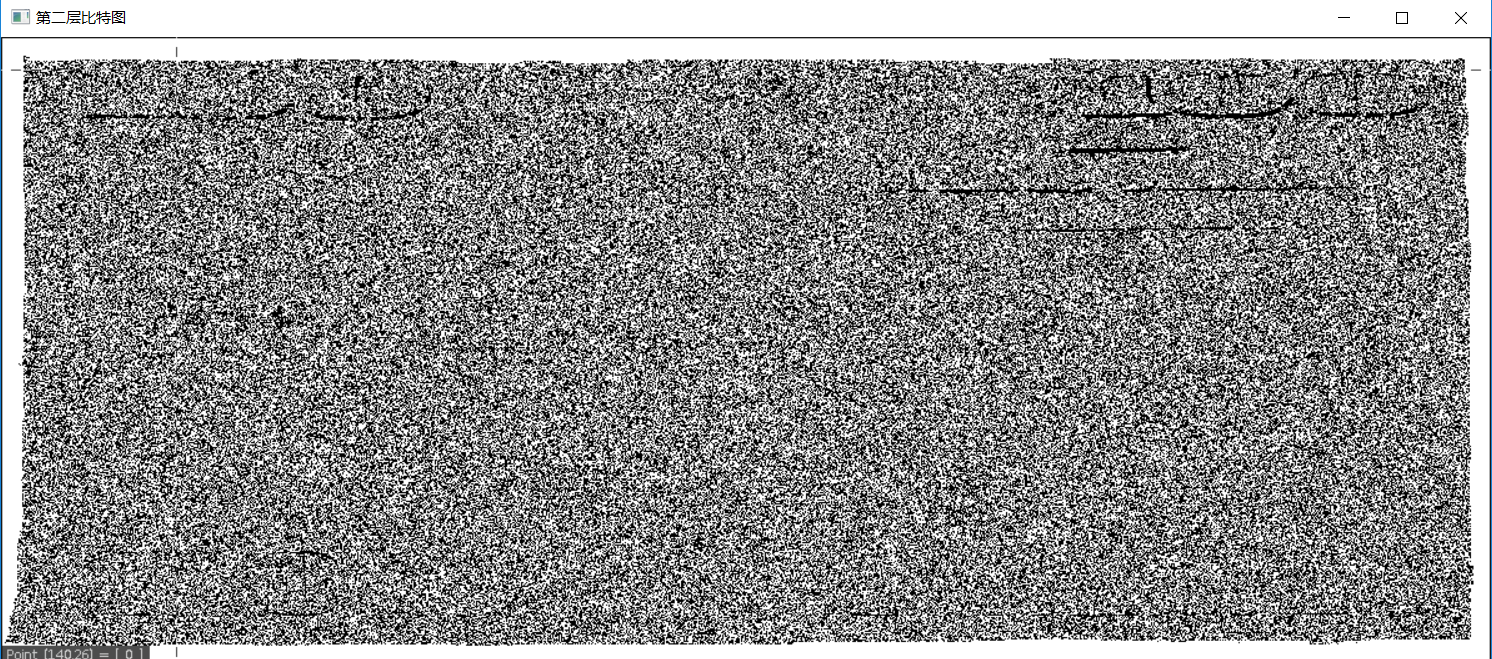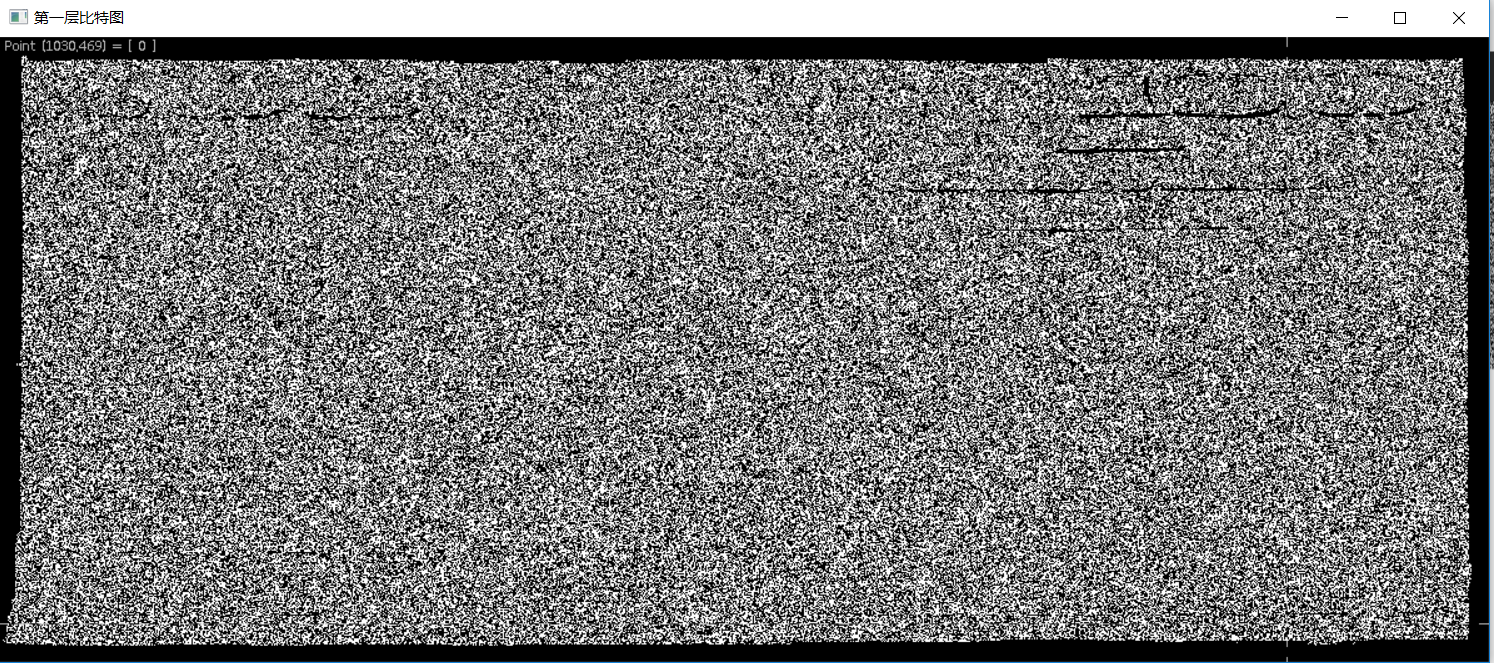### 均衡化处理

• CImg有专门显示密度分布直方图的histogram函数
	CImg<int> hist = img.histogram(256, 0, 255); //histogram的第一个参数是共有256个灰度级，第二个参数是灰度的最小值，第三个是灰度最大值
hist.display_graph();

	CImg<int> img;
CImg<int> pic = img;
img.display("处理前图片");
int size = img.size();
CImg<int> hist = img.histogram(256, 0, 255); //histogram的第一个参数是共有256个灰度级，第二个参数是灰度的最小值，第三个是灰度最大值
hist.display_graph("处理前直方图");
double sum = 0.0;
map<int, int> index;
for (int i = 0; i < 256; i++) {
sum += (double) hist(i)/size;
int Sum = (int)(sum * 255);
index.insert(pair<int, int>(i, Sum));
}
//map<int, int>::iterator iter = index.begin();
//map<int, int>::iterator end = index.end();
/*for (; iter != end; iter++) {
cout << iter->first<<":"<< iter->second << endl;
}*/
cimg_forXY(pic, x, y) {
pic(x, y) = index[pic(x, y)];
//cout << pic(x, y) << " ";
}
pic.display("处理后图片");
CImg<int> hist1 = pic.histogram(256, 0, 255);
hist1.display_graph("处理后直方图");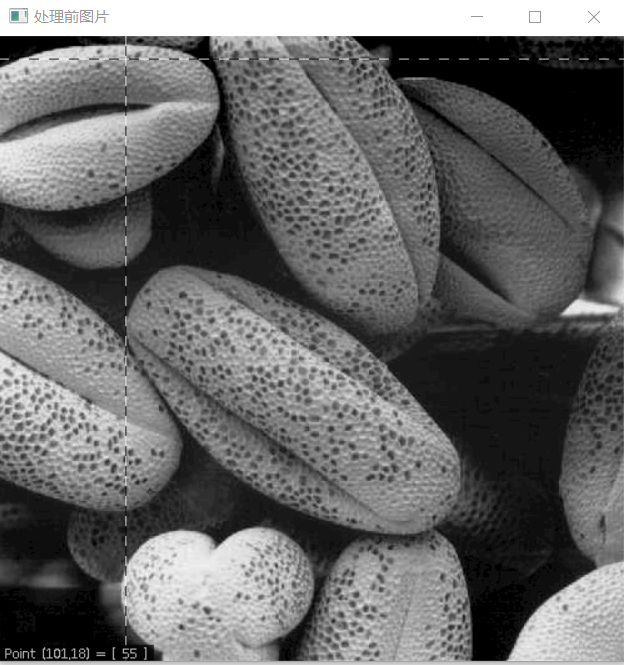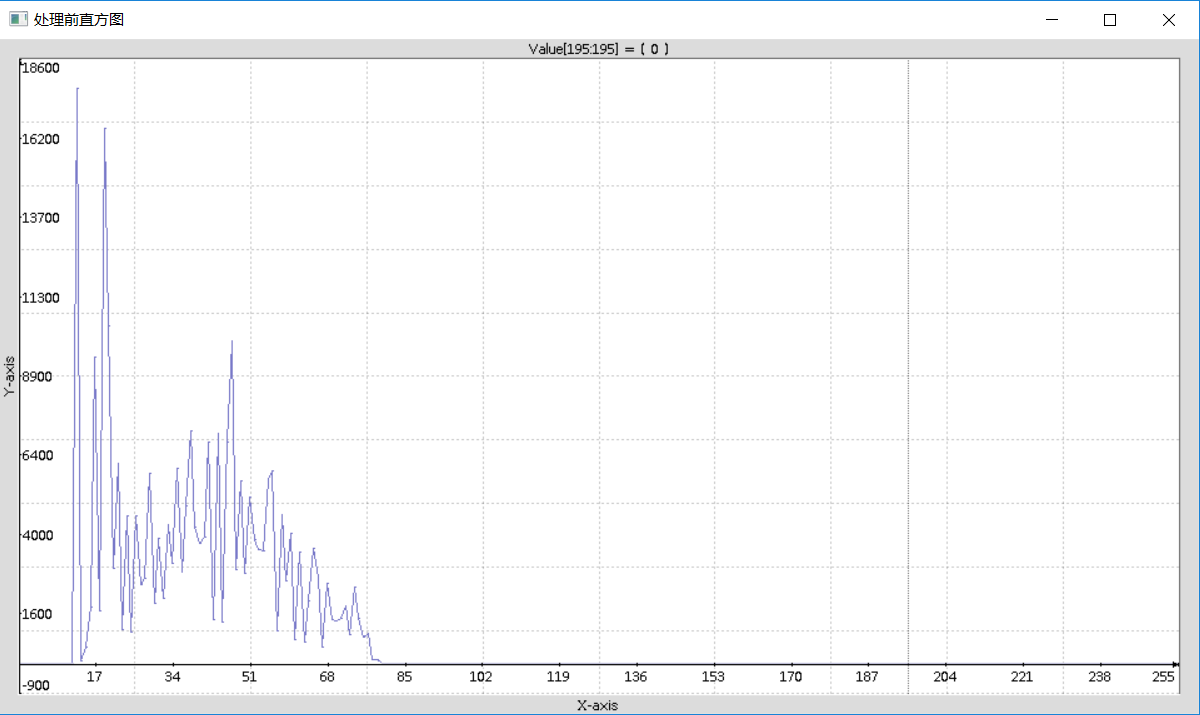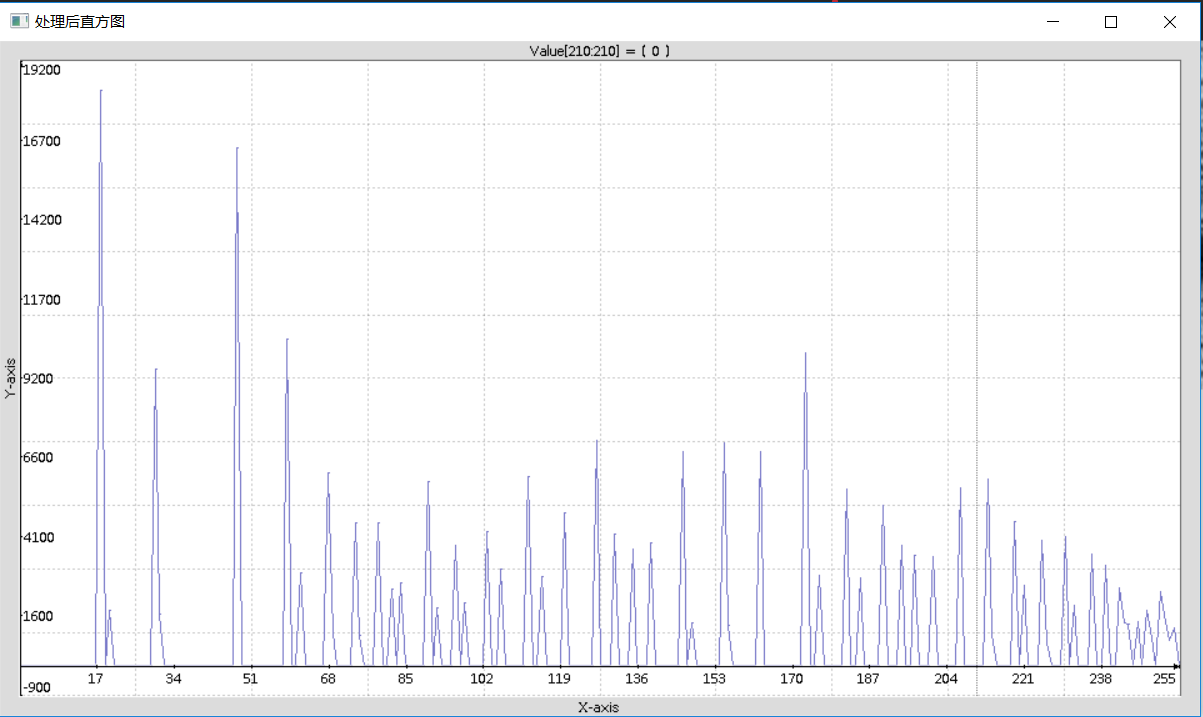2017-06-24 22:40:20 Dangkie 阅读数 6471
• ###### webgl基础篇视频教程-坚如磐石

26464 人正在学习 去看看 张立铜

1. 线性函数（反转和恒等变换）
2. 对数函数（对数和反对数变换）
3. 幂律函数（n次幂和n次根变换）r和s代表处理前后的像素值。灰度级范围[0，L-1]，如[0,255]1. 对比度拉伸
2. 灰度级分层
3. 比特平面分层：
256级灰度图像中，每个像素的灰度由8比特（一个字节）组成（281$2^8-1$）。比特平面分层可用于图像压缩，一般存储4个高阶比特平面将允许我们以可以接受的细节来重建原图像。

1. 直方图均衡

2. 直方图匹配（规定化）

- (1).其增强原理是先对原始的直方图均衡化：S = T(r)
- (2).同时对规定的直方图均衡化：v = G(z)
- (3).由于都是均衡化，故令 S = v，则：z=G1(v)=G1[T(r)]$z = G^{-1}(v) = G^{-1}[T(r)]$
3. 局部直方图处理
4. 直方图统计进行图像增强